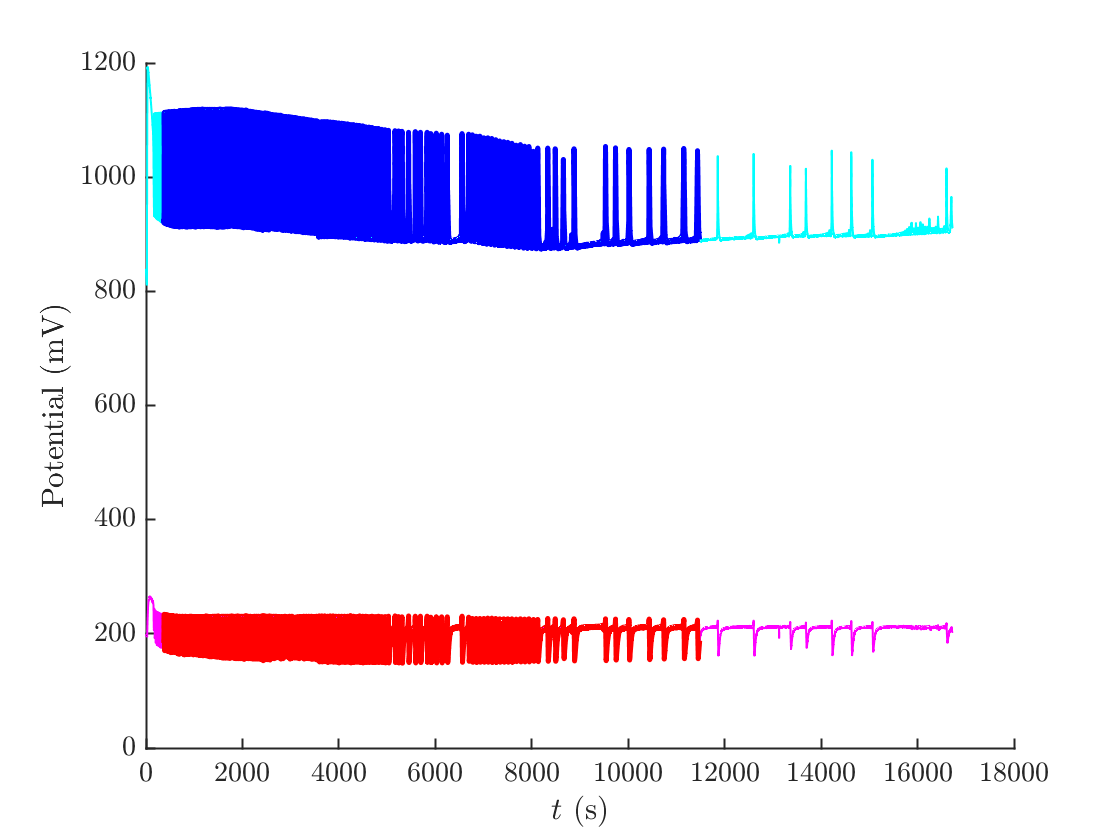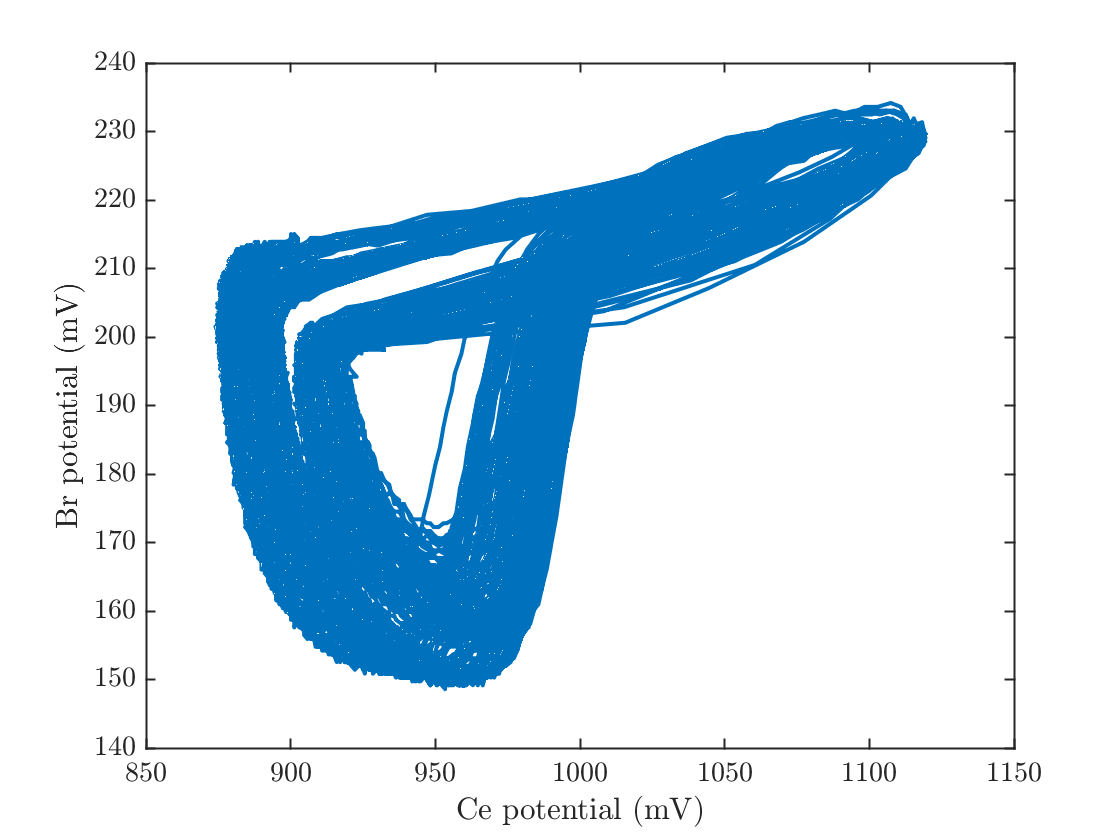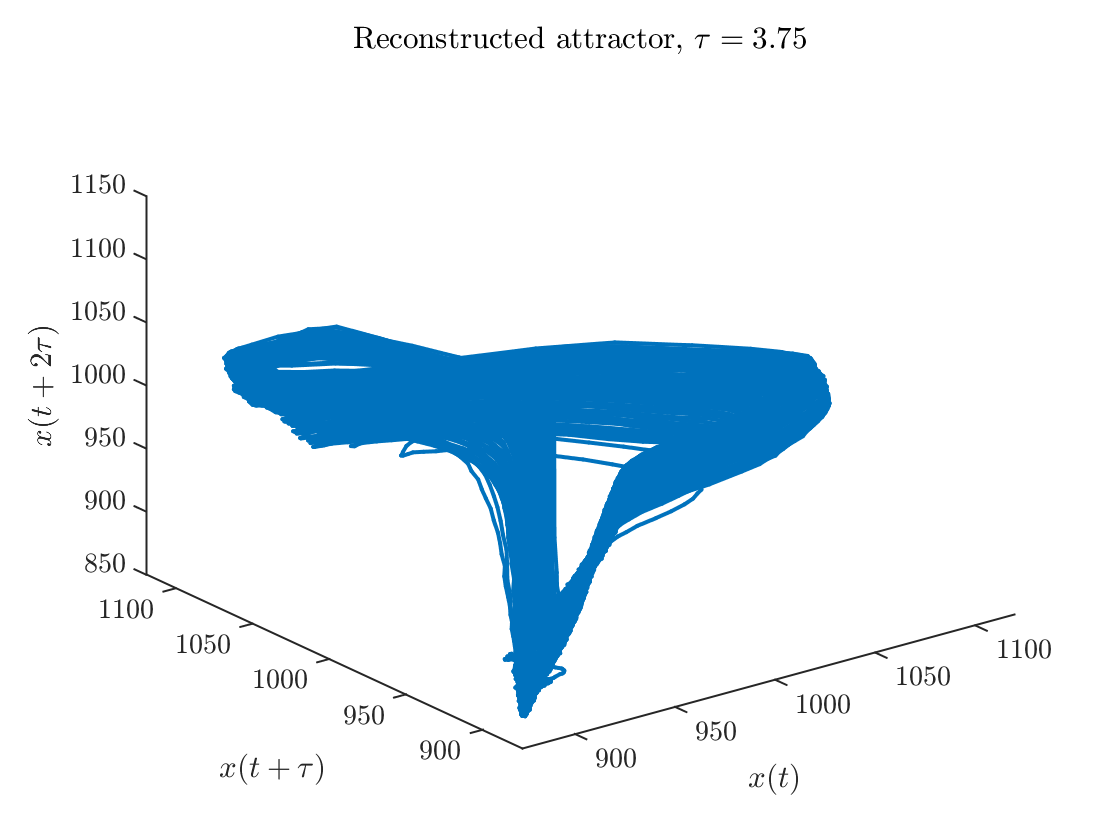# Attractor equations demo

Read in the data from the Belousov-Zhabotinsky reaction experiment. It is stored in an Excel spreadsheet. The three columns are time (in 0.25 second intervals), Cerium potential, and Bromide potential in mV.
This data was collected by students in the capstone lab in Prof. Petropoulos's section of Math 451H in Spring 2005. The data was recorded over 4.5 hours with a two channel A/D converter with probe amplifiers to get a good signal out of the probes. The reaction was conducted in a magnetically stirred beaker, but without inflow of fresh reagents nor outflow of the mixture.
tLong=BZData(:,1);CeLong=BZData(:,2);BrLong=BZData(:,3);
dt=tLong(2)-tLong(1);

## Reconstruct using time-delay embedding

Choose the start and end times and the length of the time delay.
tStart=5901; % Initial time to include in computation
tEnd =11482; % Final time to include
lag=15; % Time lag for time-delay embedding
tEnd=max(tEnd, tStart+1); % Make sure that the times make sense
tEnd=min(max(tLong), tEnd);
nStart=floor(tStart/4); % Convert times to indices
nEnd = floor(tEnd)/dt;
Cut off unused portions
t=tLong(nStart:nEnd); nt=length(t);
Ce=CeLong(nStart:nEnd);
Br=BrLong(nStart:nEnd);
Plot the potentials as a function of time
figure(1);clf; hold on;
plot(tLong,CeLong,'c','linewidth',1)
plot(t,Ce,'b','linewidth',2.5)
plot(tLong,BrLong,'m','linewidth',1)
plot(t,Br,'r','linewidth',2.5)
xlabel('$t$ (s)')
ylabel('Potential (mV)')
hold offPlotting the two potentials against each other show that any attractor must be greater than 2-dimensional, since the trajectory appears to self-intersect.
figure(2);
plot(Ce,Br)
xlabel('Ce potential (mV)')
ylabel('Br potential (mV)')Compute the time-delay embedding in three dimensions. Here we use the Cerium potential but the Bromide potential should work equally well.
dim=3;
ps=zeros(nEnd-nStart-dim*lag+1,3);
for i=1:dim
firstpoint=1+(i-1)*lag;
lastpoint=nt-(dim-(i-1))*lag;
ps(:,i)=Ce(firstpoint:lastpoint);
end
Plot the reconstructed attractor
figure(3)
plot3(ps(:,1),ps(:,2),ps(:,3))
xlabel('$x(t)$'); ylabel('$x(t+\tau)$');zlabel('$x(t+2\tau)$')
title(sprintf('Reconstructed attractor, $\\tau=%0.2f$',lag*dt))